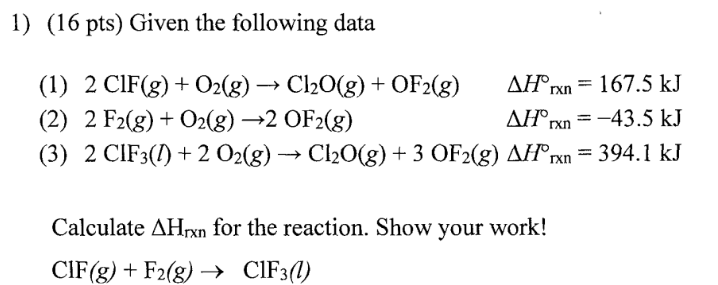Chemistry Practice Problems Hess's Law Practice Problems Solution: Given the following data (1) 2ClF (g) + O2 (g) → C...

🤓 Based on our data, we think this question is relevant for Professor Ratliff's class at USF.

# Solution: Given the following data (1) 2ClF (g) + O2 (g) → Cl2O (g) + OF2 (g)         ΔH°rxn = 167.5 kJ (2) 2F2 (g) + O2 (g) → 2OF2 (g)                          ΔH°rxn = -43.5kJ (3) 2ClF3 (l) + 2O2 (g) → Cl2O (g) + 3OF2 (g)    ΔH°rxn = 394.1 kJ Calculate ΔHrxn for the reaction. Show your work! ClF (g) + F2 (g) → ClF3 (l)

###### Problem
Given the following data

(1) 2ClF (g) + O2 (g) → Cl2O (g) + OF2 (g)         ΔH°rxn = 167.5 kJ

(2) 2F2 (g) + O2 (g) → 2OF2 (g)                          ΔH°rxn = -43.5kJ

(3) 2ClF3 (l) + 2O2 (g) → Cl2O (g) + 3OF2 (g)    ΔH°rxn = 394.1 kJ

Calculate ΔHrxn for the reaction. Show your work!

ClF (g) + F2 (g) → ClF3 (l)View Complete Written Solution

Hess's Law

Hess's Law

#### Q. A chemist measures the enthalpy change ΔH during the following reaction: CS2 (g) + 3Cl2 (g) → CCl4 (l) + S2Cl2 (l)     ΔH = -304. kJ Use this informat...

Solved • Fri Sep 14 2018 12:42:54 GMT-0400 (EDT)

Hess's Law

#### Q. A chemist measures the enthalpy change ΔH during the following reaction: P4 (s) + 6Cl2 (g) → 4 PCl3 (g)       ΔH = -1148.kJ Use this information to co...

Solved • Fri Sep 14 2018 12:37:47 GMT-0400 (EDT)

Hess's Law

#### Q. A chemist measures the enthalpy change ΔH during the following reaction: 2H2O (l) + O2 (g) → 2H2O2 (l)            ΔH = 196. kJ Use this information to...

Solved • Fri Sep 14 2018 12:23:18 GMT-0400 (EDT)

Hess's Law

#### Q. Use the following data to calculate the standard heat (enthalpy) of formation, ΔH °f, of manganese(IV) oxide, MnO2 (s). 2MnO2 (s) → 2MnO (s) + O2 (g) ...

Solved • Tue Aug 21 2018 14:24:03 GMT-0400 (EDT)